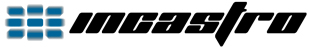Back

Android - OpenGL ES 1 - Tutorial 2 - Draw a Triangle

Consider the triangle of the following image:Continuing on the basic 2D project, create a new class "ObjTriangle" and replace the code with the following:
```package opengles1.tutorial2;

import java.nio.ByteBuffer;
import java.nio.ByteOrder;
import java.nio.FloatBuffer;
import java.nio.ShortBuffer;

import javax.microedition.khronos.opengles.GL10;

public class ObjTriangle {
private FloatBuffer vertexBuffer;
private ShortBuffer indexBuffer;

private float[] vertices = {
-0.5f, -0.5f,
0.5f, -0.5f,
0.0f, 0.5f
};

private short[] indices = {
0, 1, 2
};

public ObjTriangle() {
// Set vertex buffer from data.
ByteBuffer vbb = ByteBuffer.allocateDirect(this.vertices.length * 4);
vbb.order(ByteOrder.nativeOrder());
this.vertexBuffer = vbb.asFloatBuffer();
this.vertexBuffer.put(this.vertices);
this.vertexBuffer.position(0);

// Set index buffer from data.
ByteBuffer ibb = ByteBuffer.allocateDirect(this.indices.length * 2);
ibb.order(ByteOrder.nativeOrder());
this.indexBuffer = ibb.asShortBuffer();
this.indexBuffer.put(this.indices);
this.indexBuffer.position(0);
}

public void DrawTriangle(GL10 gl) {
// Enable client state.
gl.glEnableClientState(GL10.GL_VERTEX_ARRAY);
// Point to buffer.
gl.glVertexPointer(2, GL10.GL_FLOAT, 0, this.vertexBuffer);
// Set color for all vertex (red).
gl.glColor4f(1.0f, 0.0f, 0.0f, 1.0f);
// Draw.
gl.glDrawElements(GL10.GL_TRIANGLES, this.indices.length, GL10.GL_UNSIGNED_SHORT, this.indexBuffer);
// Disable the client state.
gl.glDisableClientState(GL10.GL_VERTEX_ARRAY);
}

}```
Now let's edit the "MainRenderer" class as follows:
```package opengles1.tutorial2;

import android.opengl.GLSurfaceView;

import javax.microedition.khronos.egl.EGLConfig;
import javax.microedition.khronos.opengles.GL10;

public class MainRenderer implements GLSurfaceView.Renderer {
int width, height;

ObjTriangle objtriangle;

// Constructor.
public MainRenderer() {
// Set class.
this.objtriangle = new ObjTriangle();
}

@Override
public void onSurfaceCreated(GL10 gl, EGLConfig config) {
gl.glClearColor(0.0f, 0.0f, 0.0f, 1.0f);
}

@Override
public void onSurfaceChanged(GL10 gl, int width, int height) {
this.width = width;
this.height = height;

gl.glViewport(0, 0, this.width, this.height);
gl.glMatrixMode(GL10.GL_PROJECTION);
gl.glOrthof(0.0f, this.width - 1.0f, 0.0f, this.height - 1.0f, 1.0f, -1.0f);

// Select model-view matrix.
gl.glMatrixMode(GL10.GL_MODELVIEW);
}

@Override
public void onDrawFrame(GL10 gl) {
gl.glClear(GL10.GL_COLOR_BUFFER_BIT);

// Translate triangle.
gl.glTranslatef(this.width / 2.0f, this.height / 2.0f, 0.0f);
// Scale triangle.
gl.glScalef(100.0f, 100.0f, 0.0f);
// Draw triangle.
this.objtriangle.DrawTriangle(gl);
}

}```
We note the presence of the constructor "MainRenderer()" as we need to define the "ObjTriangle" class when the renderer is defined in "MainView".

With "this.objtriangle = new ObjTriangle();" we call the constructor of "ObjTriangle" class where we set the data of our triangle.
The vertices of the triangle present in "vertices" and "indices" (written counterclockwise) are not in a format useful for OpenGL, in the constructor they are converted and stored in "vertexBuffer" and "indexBuffer".

In the "onSurfaceChanged()" method, after setting the projection matrix, we use the instruction "gl.glMatrixMode(GL10.GL_MODELVIEW);" to tell OpenGL that from now on we will work on the modelview matrix, all subsequent instructions will influence this matrix.

In the "onDrawFrame()" method:
- 1. clear the frame;
- 2. reset the current matrix (modelview);
- 3. translate the currente matrix;
- 4. scale the current matrix;
- 5. draw the triangle by calling the function present in the "ObjTriangle" class:
- a. enable the use of vertices;
- b. specific where the vertices are;
- c. specific the color of all the vertices (red);
- d. draw;
- e. disable the use of vertices.

The result is:During the drawing phase in "DrawTriangle()", the vertices and the position of the triangle will be influenced by the modelview matrix that has previously been translated and scaled.

Knowing that our orthogonal coordinate system is "1:1" with the screen and the triangle is "1x1", translating by 200 is equivalent to move of 200 and scaling by 10 is equivalent to a resizing of 10. But be careful of the order! If we first scale by 10, when we move by 200 we will move of 2000, that is the new "basic unit" (10) multiplied by 200.

OpenGL ES 1 Tutorial 2Back